#### Simplifying the inertia matrix

Now the inertia matrix will be considered more carefully. It is a symmetric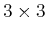matrix, which can be expressed as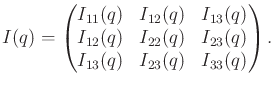(13.93)

For each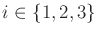, the entry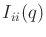is called a moment of inertia. The three cases are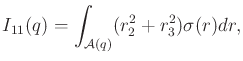(13.94)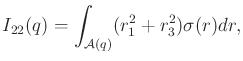(13.95)

and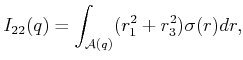(13.96)

The remaining entries are defined as follows. For each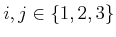such that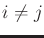, the product of inertia is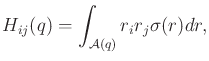(13.97)

and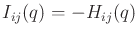.

One problem with the formulation so far is that the inertia matrix changes as the body rotates because all entries depend on the orientation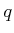. Recall that it was derived by considering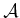as a collection of infinitesimal particles in the translating frame. It is possible, however, to express the inertia matrix in the body frame of. In this case, the inertia matrix can be denoted as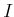because it does not depend on the orientation ofwith respect to the translational frame. The original inertia matrix is then recovered by applying a rotation that relates the body frame to the translational frame: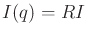, in which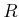is a rotation matrix. It can be shown (see Equation (2.91) and Section 3.2 of ) that after performing this substitution, (13.92) simplifies to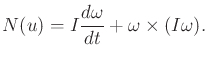(13.98)

The body frame ofmust have its origin at the center of mass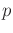; however, its orientation has not been constrained. For different orientations, different inertia matrices will be obtained. Sincecaptures the physical characteristics of, any two inertia matrices differ only by a rotation. This means for a given, all inertia matrices that can be defined by different body frame orientations have the same eigenvalues and eigenvectors. Consider the positive definite quadratic form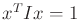, which represents the equation of an ellipsoid. A standard technique in linear algebra is to compute the principle axes of an ellipsoid, which turn out to be the eigenvectors of. The lengths of the ellipsoid axes are given by the eigenvalues. An axis-aligned expression of the ellipsoid can be obtained by defining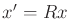, in whichis the matrix formed by columns of eigenvectors. Therefore, there exists an orientation of the body frame in which the inertia matrix simplifies to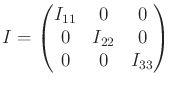(13.99)

and the diagonal elements are the eigenvalues. If the body happens to be an ellipsoid, the principle axes correspond to the ellipsoid axes. Moment of inertia tables are given in many texts ; in these cases, the principle axes are usually chosen as the axis of the body frame because they result in the simplest expression of.

Steven M LaValle 2020-08-14